Home > CC4 > Chapter 11 > Lesson 11.1.2 > Problem11-18

11-18.
1. A rumor about the school dance is being spread throughout a school. It began at 8 a.m. this morning, and each hour the fraction of the students that know the rumor triples. The principal does not know what fraction of students started the rumor. Homework Help ✎

1. What is the multiplier for the geometric sequence in this situation?

2. By 3 p.m. this afternoon, every student has heard the rumor. What fraction of students had heard the rumor at 2 p.m.?

3. Let a represent the unknown fraction of the student population that started the rumor. Write an equation for a geometric sequence that represents the fraction of students that have heard the rumor after n hours.

4. Use the fact that the every student has heard the rumor by 3 p.m. and your equation to determine the fraction of the student population that started the rumor.

5. If a group of 3 students started the rumor initially, how many students are in the school?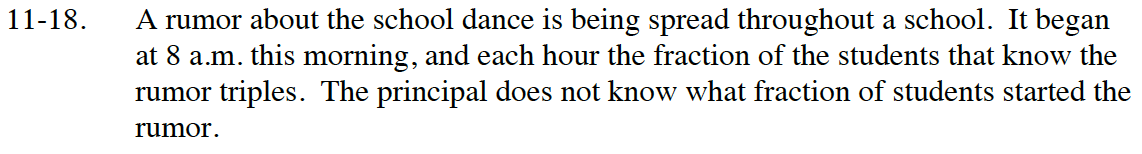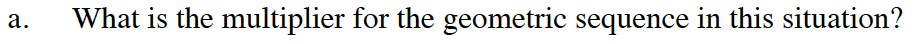How do you triple something?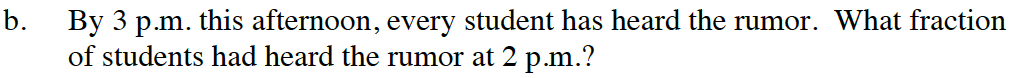Between 2 p.m. and 3 p.m., the number of people who had heard the rumor tripled so that the whole student body knows.
If the whole student body is 1, what fraction times 3 equals 1? That is, ? · 3 = 1?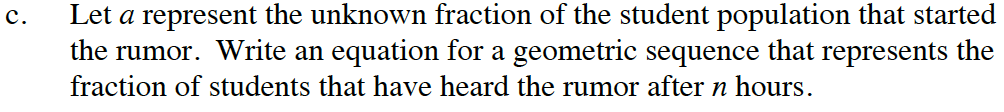a · 3n = y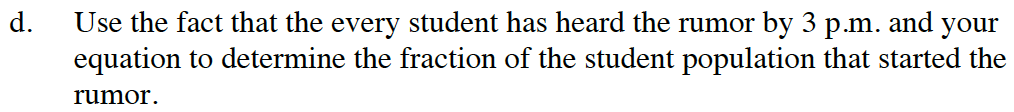Use the equation from part (c) where n is the number of hours that have passed between 8 a.m. and 3 p.m. and y is 1.

Solve the equation for a: a · 37 = 1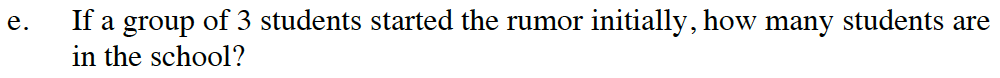Let s = the total number of students in school.

$\text{Since }a = \frac{1}{2187}, \frac{3}{s}\text{ is an equivalent fraction. That is, }\frac{1}{2187}=\frac{3}{s}.$